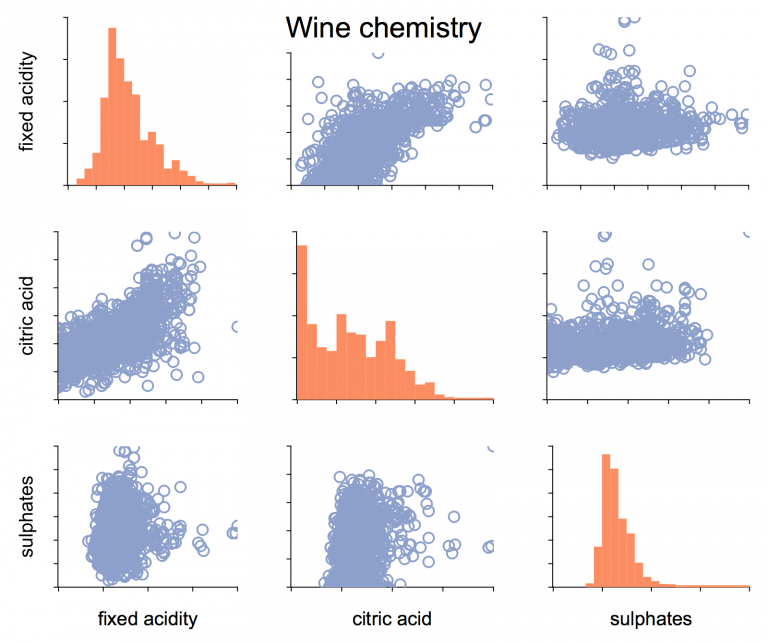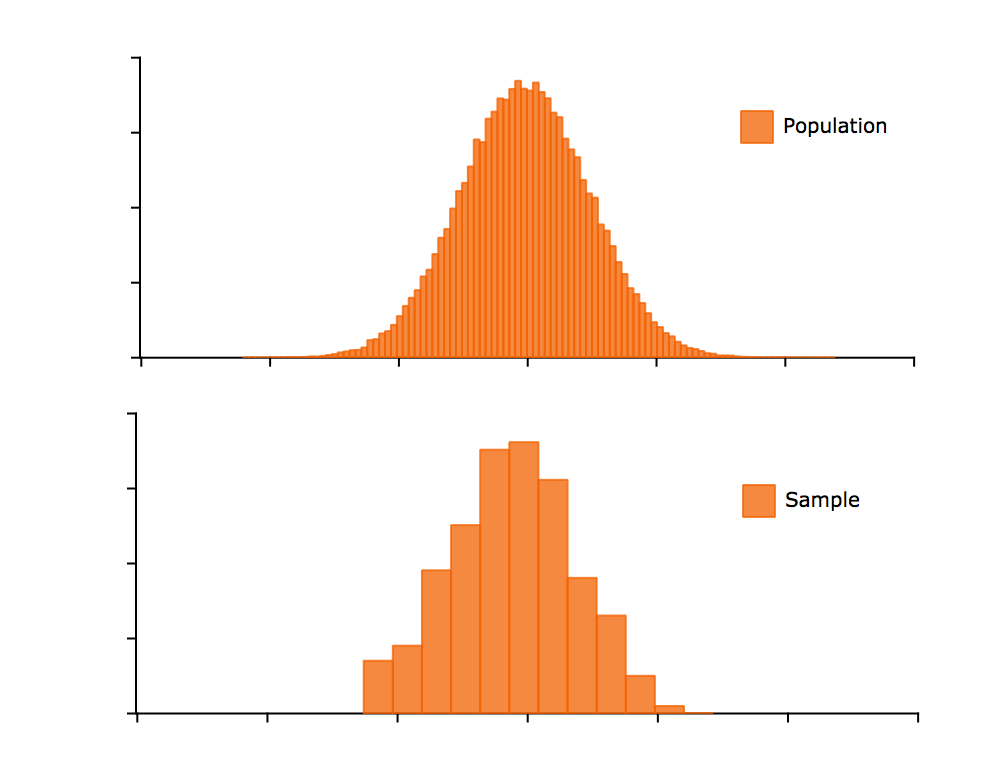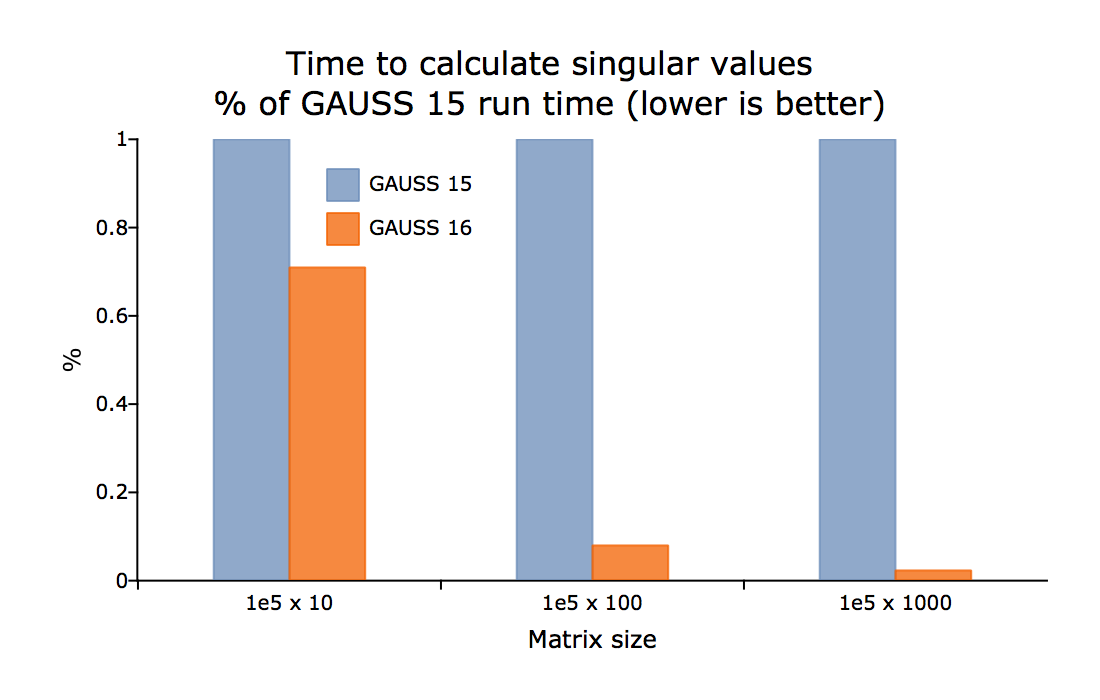What's New in GAUSS 17

What's New in GAUSS 17

Improved Data HandlingOptionen zum Festlegen von Modellen und Daten hinsichtlich Modellvariablen

//Load specified variables in a GAUSS matrix:
X = loadd("wine_quality.dat", "rating + citric acid + sulphates");

//Estimate parameters of model:
//weight = α + β1*height + β2*age

call ols("students.dat", "weight ~ height + age");

//Calculate descriptive statistics on all
//variables in dataset except for lot_size and num_baths

call dstatmt("housing.dat", ". -lot_size -num_baths");

• Simple to use
• Consistent with other statistical packages
• Well documented
• Backwards compatible

Support for HDF5 datasets provides

• Unlimited dataset size
• Fast data read and write
• Supported as native GAUSS file type
• Portable to all operating systems and many software packages

Compute and estimate CSV, XLSX and HDF5 data directly

Intelligent file handling allows you to use many different file types as data sources for GAUSS procedures:

//Load specified variables from a CSV file to a GAUSS matrix:
X = loadd("wine_quality.csv", "rating + citric acid + sulphates");

//Estimate parameters of model:
//rating = α + β1*citric acid + β2*sulphates,
//using data from an Excel file call

ols("wine_quality.xlsx", "rating ~ citric acid + sulphates");

//Calculate descriptive statistics on all variables
//in an Excel file except for 'fixed acidity' and 'chlorides'
call dstatmt("wine_quality.xlsx", ". -fixed acidity -chlorides");

New Graphics Functionality

Support for LaTeX in titles, legends, axis labels and text boxes

plotSetTitle(&myPlot, "\\Delta y = y_t - y_{t-1}");

New functions

Create XY plots with user specified symmetrical or asymmetrical error bars
Adds a surface or plane to an existing surface plot
• plotSetLegendFont:
Controls the font family, size and color of the text in the legend
• plotCDFEmpirical:
Plots the empirical distribution function of an input vector or vectors

Graphics Editor now allows interactive control of

• View angle, lighting and toggle the mesh in surface graphs
• Extent of range of X and Y axes

Function enhancements

• New color maps for surface and contour plots make it easy to create professional and attractive 3-D graphics
• Added option to place height on contour lines in plotContour
• Added option to place colors at specific heights, rather than splitting the colors evenly for surface and contour plots
• The terminal version of GAUSS, 'tgauss', and the GAUSS Engine can now create and save graphs on headless servers

GAUSS HPCC

GAUSS HPCC (High Performance Cluster Computing) boosts the computing power of GAUSS, harnessing the capabilities of high speed cluster machines, for incredible speed and performance.

Built-in, efficient cluster computing support

• Create high-level GAUSS programs that use the fast, low-level MPI library
• A version of GAUSS HPCC will be made available to Universities who own a Floating Network license with current Platinum Premier Support & Maintenance at no extra charge

Builds on these features included in the standard version of GAUSS 17

• Easily create GAUSS mapper and reducer functions
• Connect to NoSQL and Big Data databases such as:
• MongoDB, Hbase, Hive, Pig and more
• Support for streaming or online algorithms for data that does not fit entirely in memory.

New Mathematical and Statistical Functionality

New functions

• cdfEmpirical:
Computes the empirical cumulative distribution function
• ldl:
Computes and returns the 'L' and 'D' factors from a symmetric matrix
• powerm:
Raises a matrix to a specified power
• sylvester:
Calculates the solution to the Sylvester matrix equation
• rndWishartInv:
Takes draws from the Inverse Wishart distribution
• pdfWishartInv:
Computes the probability density function of the inverse Wishart distribution
• dot: Computes the dot product for a vector or group of vectors

Function Speedups

• X 'X for large matrices is nearly twice as fast
• sortc is much faster, especially for column vectors
• Greatly improved speed of unique and uniquesa, especially when operating on string arrays
• Linear solve, using the slash-operator '/' for small matrices
• Kronecker product '.*.' is faster when one of the inputs is a column vector
• crossprd is faster for case of fewer than 500 vectors
• cdffc is 10-1000x faster when 'd1' parameter is equal to one
• reclassify is much faster and uses less memory

Other Enhancements

Function enhancements

• quantile/quantiled:
Added option to specify the calculation method
• glm:
Added support for inverse Gaussian family and models without intercepts when estimating the parameters of the General Linear Model
• schur:
Added support for immediate return of complex form

What's New in GAUSS 16

New Data Import Wizard

• Reads CSV, XLS, XLSX as well as other delimited text files.
• Intuitive interface allows you to quickly preview and import your data.
• Visual feedback and color coding enhance experience.
• Handles well formed and malformed data files.

Simpler data preparation - Data reclassification, recoding and scaling

Reclassification and recoding

GAUSS 16 comes with new functions that make it easy to transform categorical variables from text labels to numeric labels, numeric labels back to text labels, or place numeric ranges into separate categories.

1. The first function is reclassify. You can use it to:
• Reclassify text labels to numeric category labels.
• Reclassify numeric labels to text labels.
• Reclassify vectors individually, an entire matrix or a multidimensional array.
Reclassify text labels to numeric categories
//Create a 7x1 string vector
X = "EU" \$| "GBP" \$| "USD" \$|
"GBP" \$| "USD" \$| "EU" \$| "EU";

//Use 'uniquesa' to create a string vector
//with the unique strings in 'X' listed
//in alphabetical order
from = uniquesa(X);

//Create 3x1 vector of numeric category labels
to = { 0, 1, 2 };

//Reclassify elements in 'X' from
// EU -> 0
// GBP -> 1
// USD -> 2
X_numeric = reclassify(X, from, to);
1. The second new function is reclassifyCuts, which
• Places data into numeric categories based upon range.
• Allows intervals to be open or closed on the right.
• Takes vector, matrix or multidimensional array inputs.

Data scaling

One of the most common reasons for a maximum likelihood estimation or optimization routine to fail is poorly scaled data. The new function, rescale gives you 8 different scaling options with one simple line of code. You can either:

• Use a named method and return the data plus scaling factors
//Scale each column of 'x_train'
{ x_train, location, scale } = rescale(x_train, standardize");
• The location and scale passed in later to scale another sample from the same data set:
//Scale each column of 'x_test' with scale and
//location parameters created from training data above
x_test = rescale(x_test, location, scale);

New Sampling functionsNew Sampling Functions in GAUSS 16

Sample without replacement:

//Take a 100 observation sample from 'x'
//without replacement
sample = sampleData(x, 100);

Sample with replacement:

replace = 1;
//Take a 100 observation sample from 'x'
//WITH replacement
sample = sampleData(x, 100, replace);

Create training set and test set.

n = rows(x);

//Create indices for training set
idx_train = sampleData(seqa(1,1,n), 0.75 * n);

//Extract training set
x_train = x[idx_train];

//Remove (or delete) training set rows from 'x'
//to create test set
x_test = delrows(x, idx_train);

Create random indices to draw from multiple variables:

//Create random integers from between 1 and 1000
range = { 1, 1000 };
idx = rndi(50, 1, range);
//Sample same observations from 'x' and 'y'
x_sample = x[idx,.];
y_sample = y[idx];

Generalized Linear Model

In statistics, the generalized linear model (GLM) is a flexible generalization of ordinary linear regression that allows for response variables that have error distribution models other than a normal distribution. The GLM generalizes linear regression by allowing the linear model to be related to the response variable via a link function and by allowing the magnitude of the variance of each measurement to be a function of its predicted value.

The GAUSS function glm is used to solve generalized linear model problems. GAUSS provides the following combinations from exponential family and related link function.

Normal Binomial Poisson Gamma
identity * * * *
inverse * * * *
ln * * * *
logit   *
probit   *

Format

// Read data matrix from a '.csv' file and start from the second row

// Specify dependent variable
y = data[.,1];

// Specify independent variable
x = data[.,2:4];

// Call glm function
call glm(y, x, "binomial", vnames, 3, link);

Output

Generalized Linear Model

Valid cases: 400 Dependent Variable: admit
Degrees of freedom: 394 Distribution: binomial
Pearson Chi-square: 397.5 AIC: 470.5
Log likelihood: -229.3 BIC: 494.5
Dispersion: 1 Iterations: 4

Standard Prob
Variable Estimate Error z-value >|z|
---------------- ------------ ------------ ------------ ------------
CONSTANT -3.99 1.14 -3.5001 0.000465027
rank 2 -0.67544 0.31649 -2.1342 0.0328288
3 -1.3402 0.34531 -3.8812 0.000103942
4 -1.5515 0.41783 -3.7131 0.000204711
gre 0.0022644 0.001094 2.0699 0.0384651
gpa 0.80404 0.33182 2.4231 0.0153879

Note: Dispersion parameter for BINOMIAL distribution taken to be 1

Simplified function calls

Pass in only the arguments you need

Many GAUSS procedures used to require passing in all arguments including control structures. Additionally many GAUSS procedures that call a user-defined procedure, such as optimization or integration functions used to require extra data to be passed in as a DS structure.

• Question: Will this require me change my code?

No! It is completely backwards compatible.

• Question: Does this remove any of the options or flexibility?

No! The control structures and all of their options remain available, you just do not need to use them for cases in which you would use the default settings.

We will use the new GAUSS function, integrate1d with a toy example to illustrate the differences.

Old style

//Define procedure to be integrated
proc (1) = myProc(x, struct DS d);
local y;
y = d.dataMatrix;
retp(exp( -(x .* x) / (2 .* y) ));
endp;

//Define limits of integration
x_min = -1000;
x_max = 1000;

//Define extra argument for procedure 'myProc'
struct DS d;
d = dsCreate();
d.datamatrix = 3;

//Define 'ctl' to be a control structure
struct integrateControl ctl;

//Fill in with default values
ctl = integrateControlCreate();

//Calculate integral
integral = integrate1d(&myProc, x_min, x_max, d, ctl);

New simpler style

//Define procedure to be integrated
proc (1) = myProc(x, z);
retp(exp( -(x .* x) / (2 .* z) ));
endp;

//Define limits of integration
x_min = -1000;
x_max = 1000;

//Define extra arguments for procedure 'myProc'
a = 3;

//No need for control structure if using default values
integral = integrate1d(&myProc, x_min, x_max, a);

Speed ups and new functions

SpeedupsSpeedup in GAUSS 16 (Gallery - click to zoom and browse)

New Functionality

• QZ decomposition with options to sort the eigenvalues (qz)
• Hypergeometric CDF, PDF and random number generation (cdfHyperGeo,pdfHyperGeo, rndHyperGeo).
• Binomial PDF and Poisson PDF (pdfBinomial, pdfPoisson).
• Option to sort eigenvalues of generalized schur decomposition (qz).
• More powerful and easy to use integration function, using adaptive quadrature (integrate1d).
• Function to set axes line color and thickness (plotSetAxesPen).
• Option to specify range of random integers created by rndi.
• Option to specify delimiter for strsplit.
• Data sampling function, (sampleData).
• Data scaling function, (rescale).
• New functions for reclassifying data based upon a match (string or numeric) or a range, (reclassify, reclassifyCuts).
• Generalized linear model (glm).
• Much improved, faster and simpler to use functions for reading CSV and other delimited text files (csvReadM, csvReadSA).

User interface and other enhancements

• Syntax highlighting and brace-matching in program input/output window
• Debugger page supports file editing, 'find usages' and full editor functionality
• Improved file associations on Mac
• Bug fixes

What's New in GAUSS 15

GAUSS 15 offers many user interface improvements which make working with GAUSS easy and more comfortable. Furthermore GAUSS 15 introduces new mathematical and statistical functions and speed improvements.

User Interface

1. Enhanced 2D graphics
• Improved quality XY, polar, bar, histogram, time series and box plots.
• New area charts
• More detailed control over graph attributes
• Control over scene settings and size for exporting/printing
• Print preview for all graphs
2. Add graph objects programmatically from GAUSS
• Add shapes, lines, arrows, text boxes
• More detailed control over object attributes
3. New support for multiple graphics profiles
Create unlimited custom graph profiles throgh a simple GUI interface
4. Improved symbol editor
• Speed improvements for viewing large symbols
• More intuitive for for navigating structs and arrays
5. Improved project view
• New quick-search for finding files
• User-defined filtering options to show desired file types
6. Improved development workflow
Find/replace updated and now supports replacement in selected text.

Math and Statistical Functions

1. Improved multi-dimensional array support
• Drop down menu to select dimensions
• Right and left arrow button to traverse array dimensions
2. New random number generators
• SOBOL and Niederreiter random sequence generator
• Chi-Square and non-centric chi-squared random numbers
3. New functions, including
• Schur factoriztion with ordering of eigenvalues
• LDL factorization and solver for LDL factorized matrix

More

• New parallel for loops to increase looping speed
• Support for Retina Displays on Mac